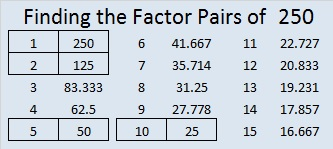# 250 and Level 5

• 250 is a composite number.
• Prime factorization: 250 = 2 x 5 x 5 x 5, which can be written 250 = 2 x (5^3)
• The exponents in the prime factorization are 1 and 3. Adding one to each and multiplying we get (1 + 1)(3 + 1) = 2 x 4 = 8. Therefore 250 has 8 factors.
• Factors of 250: 1, 2, 5, 10, 25, 50, 125, 250
• Factor pairs: 250 = 1 x 250, 2 x 125, 5 x 50, or 10 x 25
• Taking the factor pair with the largest square number factor, we get √250 = (√10)(√25) = 5√10 ≈ 15.811Print the puzzles or type the factors on this excel file: 10 Factors 2014-09-29This site uses Akismet to reduce spam. Learn how your comment data is processed.